# Calculate Angle

The calculate angle module provides a way to easily calculate the angle between two lines defined by three points. The three points are assumed to already be accessible as RoboRealm variables. The result will be placed into the result variable.

Be wary of the resulting range as the angle is calculated relative to the ordering of the points that define the lines. Due to this the resulting angle might "take the long way around". For example, an angle at 120 degrees counterclockwise is also -240 degrees clockwise. See the examples below that illustrate more of this point.

## Interface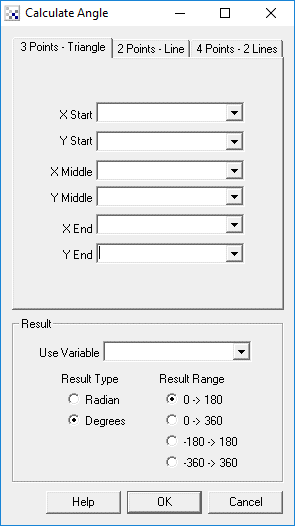## Instructions

1. 3 Points Triangle - specify the points that make up a corner. The angle measured will be the smaller angle created by the three points.

2. 2 Points Line - specify two points that make up a line. The angle measure will be the angle of this line with horizontal right being 0 deg and up being 90 deg, etc. Note that due to line symmetry 180 and 0 are the same, likewise 270 and 90, etc.

3. 4 Points 2 Lines - specify four points that describe two lines that intersect. The angle will then be the smaller angle formed by the intersection of these two lines. The larger angle would then be (360 - (2*angle))/2.

4. Result - specify the resulting variable that should hold the calculated angle, whether that measurement should be provided in radians or degrees and what range that measurement should have. Note that if you specify 0 ->180 then only acute angles are provided by disregarding the ordering of the points. If you wish to measure the angle taking in consideration the ordering of the specified points select a range that extends the result such as -360 to 360.

## ExampleClick here to load a robofile that you can use to alter the resulting angle by clicking in the main RoboRealm interface.

The following shows the output of the example file using a right triangle. Note that only the result range is changed in each image to show the different interpretations that occur when measuring an angle.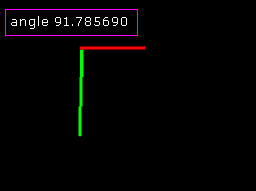Range is 0 -> 180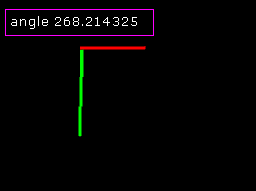Range is 0 -> 360Range is -180 -> 180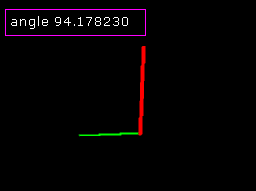Range is 0 -> 180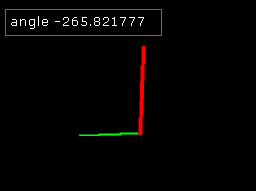Range is -360 -> 360

 Calculate_Angle Related Forum Posts Last post Posts Views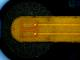Problems with Blob Tracker and with VBScript Hi STeven, I have enclosed the filtering I'm having issues with. The program should do the followin... 4 year 3 2532 Turning an Angle into a compass heading I have a downward looking camera looking at a target and have the robot gps heading,Roborealm has calculated the angle to the ta... 9 year 4 2480Calculate angle I am using the calculate angle module to calculate a 2 point line. My start point is the center of the image and t... 9 year 2 2532Load image problem Hi! I have many images, in which i need define an angle between two points (blobs) images and .robo... 10 year 7 3471 Angle between two blobs I found how I was supposed to get the values out of the arrays! :-) from this post: ... 11 year 2 2845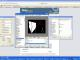Array values Short Question: I Have 2 Line probe. How do I get the values (Array) i... 11 year 2 3114Line Probe- How to use 2 Line probe to measure angle automatically How can i use line probe to measure angle between 2 line? I had detected 2 lines and i wish to use it to calculate the angle aut... 12 year 2 3228Define angle with small soccer plates Hi, can we find the angle between colors used on plates in small soccer leagues? thanks... 13 year 6 3700